# Chapter 2. Seki Takakazu

## Column Why doeshold true? (Level 3)

With respect to angle α, assume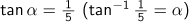.

Then, we obtain.

By repeating this, we obtain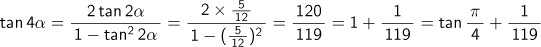.

And now, as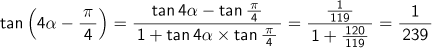holds true due to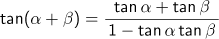,

the Addition Theorem with respect to tan,or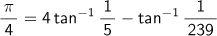is derived.

J. Machin, an astronomer from London, discovered that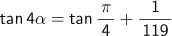held true when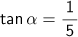, and he calculated Pi to 100 decimal points in 1706 by using this relationship and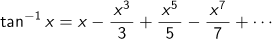.

When we actually calculate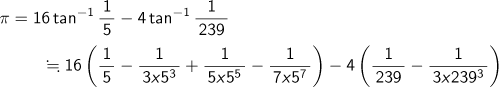with an 8-digit calculator, we obtain a figure of 3.1415918.

Subsequently, relational expressions were discovered including the following: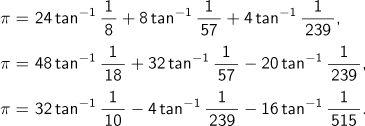Using such expressions, calculations to evaluate Pi to still further decimal places were attempted.

Top of page